# Samacheer Kalvi 10th Maths Solutions Chapter 3 Algebra Ex 3.17

You can Download Samacheer Kalvi 10th Maths Book Solutions Guide Pdf, Tamilnadu State Board help you to revise the complete Syllabus and score more marks in your examinations.

## Tamilnadu Samacheer Kalvi 10th Maths Solutions Chapter 3 Algebra Ex 3.17

10th Maths Exercise 3.17 Answers Question 1.
If A = $$\left[\begin{array}{cc}{1} & {9} \\ {3} & {4} \\ {8} & {-3}\end{array}\right]=\left[\begin{array}{ll}{5} & {7} \\ {3} & {3} \\ {1} & {0}\end{array}\right]$$ then verify that
(i) A + B = B + A
(ii) A + (-A) = (-A) + A = 0
Solution: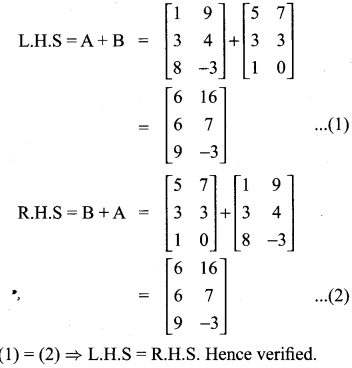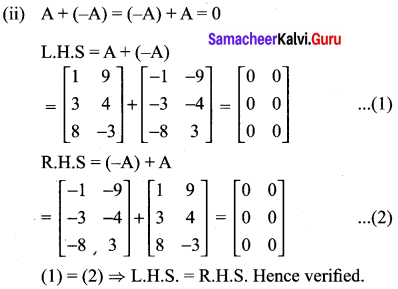10th Maths Exercise 3.17 Question 2.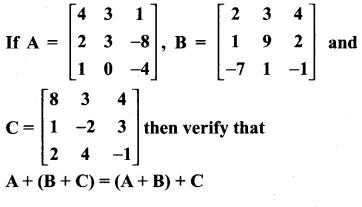Solution: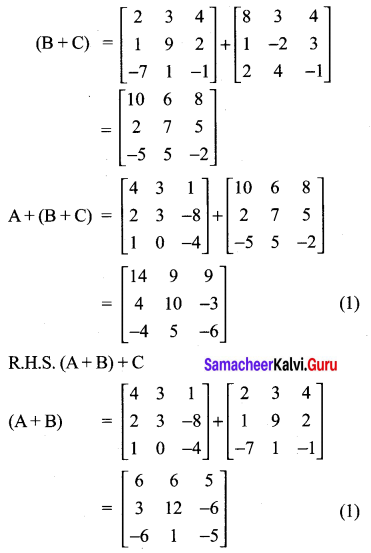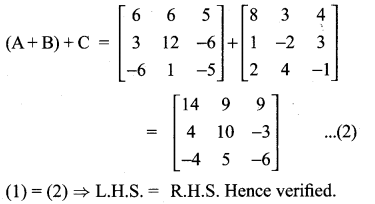Ex 3.17 Class 10 Question 3.
Find X and Y if X + Y = $$\left[\begin{array}{ll}{7} & {0} \\ {3} & {5}\end{array}\right]$$ and X – Y = $$\left[\begin{array}{ll}{3} & {0} \\ {0} & {4}\end{array}\right]$$
Solution: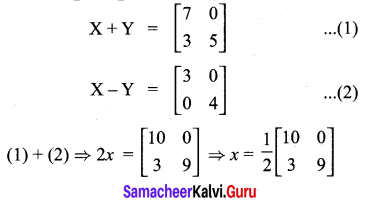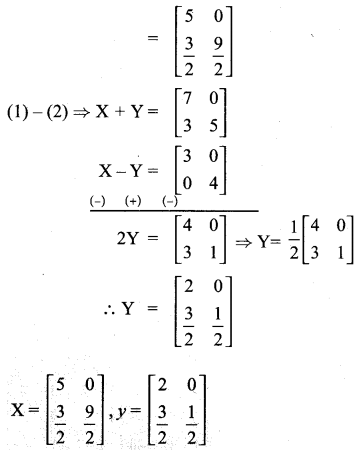10th New Syllabus Maths Exercise 3.17 Question 4.
If A = $$\left[\begin{array}{lll}{0} & {4} & {9} \\ {8} & {3} & {7}\end{array}\right]$$, B = $$\left[\begin{array}{lll}{7} & {3} & {8} \\ {1} & {4} & {9}\end{array}\right]$$ find the value of
(i) B – 5A
(ii) 3A – 9B
Solution: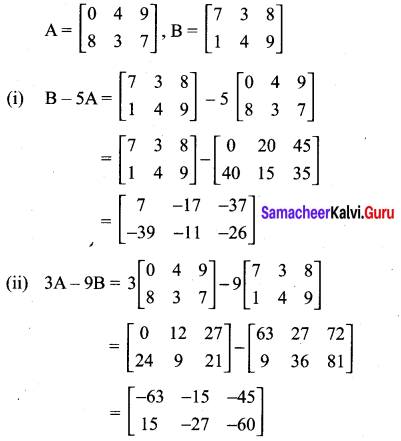Exercise 3.17 Class 10 Question 5.
Find the values of x, y, z if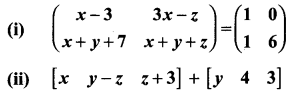Solution:
(i) $$\left(\begin{array}{cc}{x-3} & {3 x-z} \\ {x+y+7} & {x+y+z}\end{array}\right)=\left(\begin{array}{ll}{1} & {0} \\ {1} & {6}\end{array}\right)$$
x – 3 = 1 ⇒ x = 4
3x – z = 0
3(4) – z = 0
-z = -12 ⇒ z = 12
x + y + 7 = 1
x + y = -6
4 + y = -6
y = -10
x = 4, y = -10, z = 12

(ii) $$\left[\begin{array}{ccc}{x} & {y-z} & {z+3}\end{array}\right]+\left[\begin{array}{lll}{y} & {4} & {3}\end{array}\right]=\left[\begin{array}{lll}{4} & {8} & {16}\end{array}\right]$$
x + y = 4 ……………. (1)
y – z + 4 = 8 ………….. (2)
z + 3 + 3 = 16 ………….. (3)
From (3), we get z = 10
From (2), we get y – 10 + 4 = 8
From (2), we get y = 14
From (1) we get x + 14 = 4
x = -10
x = -10, y = 14, z = 10

10th Maths Exercise 3.17 Answers 2021 Question 6.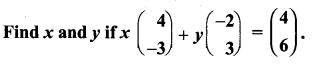Solution: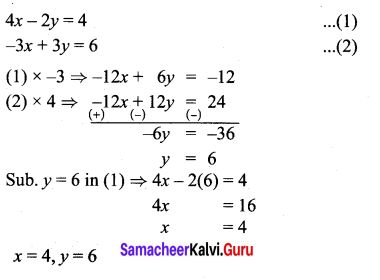10th Maths Exercise 3.17 Samacheer Kalvi Question 7.
Find the non-zero values of x satisfying the matrix equation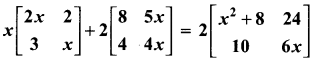Solution: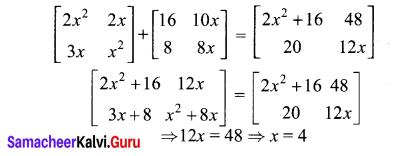Exercise 3.17 Question 8.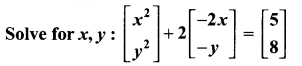Solution:
x2 – 4x = 5
y2 – 2y = 8
y2 – 2y – 8 = 0
(y – 4)(y + 2) = 0
y = 4, -2
x2 – 4x – 5 = 0
(x – 5)(x + 1) = 0
x = 5, -1
x = -1, 5, y = 4, -2